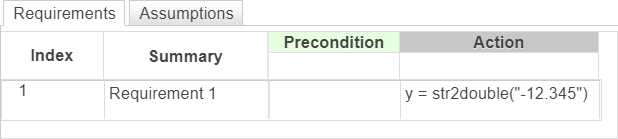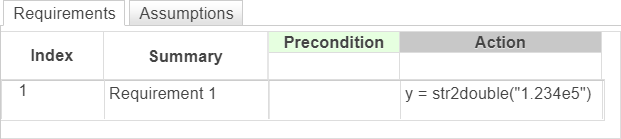# str2double, double

Convert string to double-precision value

Since R2022b

## Syntax

``X = str2double(str)``
``X = double(str)``

## Description

example

````X = str2double(str)` converts the text in string `str` to a double-precision complex value. If `str2double` cannot convert the text to a number, it returns a `NaN` value. Use this operator in the Requirements Table block.```
````X = double(str)` is an alternative way to execute `str2double(str)`.```

## Examples

expand all

In a Requirements Table block, convert the string `"-12.345"` to a double and output the value.

`y = str2double("-12.345")`In a Requirements Table block, convert the string `"1.234e5"` to a double and output the value.

`X = str2double("1.234e5")`## Input Arguments

expand all

Input value, specified as a string scalar.

`str` must contain text that represents a number, including:

• Digits

• A decimal point

• A leading `+` or `-` sign

• An `e` preceding a power of 10 scale factor

• An imaginary part followed by an `i` or a `j`

Enclose literal string with double quotes.

Data Types: `string`

## Output Arguments

expand all

Output number, returned as a double-precision complex scalar.

## Limitations

• This operator does not support the use of `Simulink.Bus` object fields.

## Version History

Introduced in R2022b